# Word Problems based on Three Sets## Cardinality Relations of Three Sets

Let, U be the universal set. A, B and C are three overlapping sets which are subsets of the universal set U.

********************

10 Math Problems officially announces the release of Quick Math Solver, an Android App on the Google Play Store for students around the world.

********************

Look at the following Venn diagram and learn the cardinal representation for the different portions.n(A) = Number of elements in set A

n(B) = Number of elements in set B

n(C) = Number of elements in set C

no(A) = Number of elements in set A only

no(B) = Number of elements in set B only

no(C) = Number of elements in set C only

n(A∩B) = Number of elements common in set A and B

n(A∩C) = Number of elements common in set A and C

n(B∩C) = Number of elements common in set B and C

no(A∩B) = Number of elements common in set A and B only

no(A∩C) = Number of elements common in set A and C only

no(B∩C) = Number of elements common in set B and C only

n(A∩B∩C) = Number of elements common to A, B and C

n(ABC) = Number of elements belongs to at least one set A, B or C.

n(A∩B∩C)c = Number of elements does not belong to the set A, B or C.

### Formula on Cardinality Relations of Three Sets

If A, B and C are subsets of universal set U then,

1.        n(ABC) = n(A) + n(B) + n(C) – n(A∩B) – n(B∩C) – n(A∩C) + n(A∩B∩C)

2.        n(A∩B∩C) = n(ABC) – n(A) – n(B) – n(C) + n(A∩B) + n(B∩C) + n(A∩C)

3.        n(ABC)c = n(U) – n(ABC)

4.        no(A) = n(A) – n(A∩B) – n(A∩C) + n(A∩B∩C)

5.        no(B) = n(B) – n(A∩B) – n(B∩C) + n(A∩B∩C)

6.        no(C) = n(C) – n(A∩C) – n(B∩C) + n(A∩B∩C)

7.        no(A∩B) = n(A∩B) – n(A∩B∩C)

8.        no(B∩C) = n(B∩C) – n(A∩B∩C)

9.        no(A∩C) = n(A∩C) – n(A∩B∩C)

10.   Exactly two = no(A∩B) + no(B∩C) + no(A∩C)

11.   Exactly one = no(A) + no(B) + no(C)

12.   At least one = n(ABC)

13.   All three = n(A∩B∩C)

14.   None of them = n(ABC)c### Word Problems Based on Three Sets

#### Workout Examples

Example 1: P, Q and R are subsets of a universal set U. If n(U) = 390, n(P) = 210, n(Q) = 165, n(R) = 120, n(P∩Q) = 60, n(Q∩R) = 45, n(A∩R) = 54 and n(P∩Q∩R) = 24, illustrate this information in a Venn-diagram and find the following:

a.        no(P)

b.        no(Q)

c.        no(R)

d.        no(P∩Q)

e.        no(Q∩R)

f.         no(P∩R)

g.        n(PQR)c

Solution:

Here,

Venn-diagram,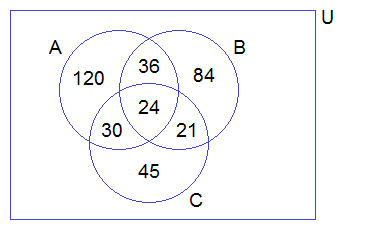From the Venn-diagram above,

a.        no(P) = 120

b.        no(Q) = 84

c.        no(R) = 45

d.        no(P∩Q) = 36

e.        no(Q∩R) = 21

f.         no(P∩R) = 30

g.        n(PQR)c = 30

Example 2: In a survey of a group of people, 60 liked tea, 45 liked coffee, 30 liked milk, 25 liked coffee as well as tea, 20 liked tea as well as milk, 15 liked coffee as well as milk and 10 liked all three. How many people were asked this question? Solve by using Venn-diagram.

Solution:

Let C, T and M represent the set of people who liked coffee, tea and milk respectively. Then,

n(T) = 60

n(C) = 45

n(M) = 30

n(C∩T) = 25

n(T∩M) = 20

n(C∩M) = 15

n(C∩T∩M) = 10

Venn diagram,Now,

n(TCM)

= n(T) + n(C) + n(M) – n(C∩T) – n(T∩M) – n(C∩M) + n(C∩T∩M)

= 60 + 45 + 30 – 25 – 20 – 15 + 10

= 85

Hence, the required number of people = 85.

Example 3: In an examination, 40% of candidates passed in mathematics, 45% in Science, and 55% in Health. If 10% passed in Mathematics and Science, 20% in Science and Health and 15% in Health and Mathematics,

(i)     Illustrate the above information by drawing a Venn diagram.

(ii)  Find the pass percentage in all three subjects.

Solution:

Let M, S, and H denote the set of students who passed in Maths, Science and Health respectively.

n(U) = 100% = n(MSH)

n(M) = 40%

n(S) = 45%

n(H) = 55%

n(M∩S) = 10%

n(S∩H) = 20%

n(H∩M) = 15%

Since, there is  no one who failed in all three subjects,

n(MSH) = n(U) = 100%

(i)        Venn-diagram,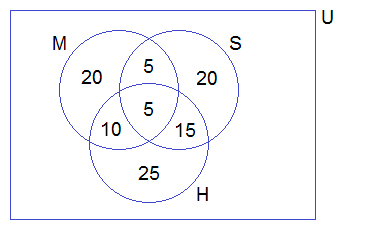(ii)     n (M∩S∩H)

= n(MSH) – n(M) – n(S) – n(H) + n(M∩S) + n(S∩H) + n(M∩H)

= 100 – 40 – 45 – 55 + 10 + 20 + 15%

= 5%

5% passed in all three subjects.

Example 4: Out of 1350 candidates, 600 passed in Science, 700 in Mathematics, 350 in English and 50 failed in all three subjects. If 200 passed in Science and Mathematics, 150 in Science and English, 100 in Mathematics and English,

(i)     How many candidates passed in all three subjects?

(ii)  Illustrate the above information in a Venn-diagram.

Solution:

Let, the set of candidates who passed in Science, Mathematics and English be S, M, and E.

n(U) = 1350

n(S) = 600

n(M) = 700

n(E) = 350

n(SME)c = 50

n(S∩M) = 200

n(M∩E) = 100

n(S∩E) = 150

We know,

n(SME)

= n(U) – n(SME)c

= 1350 – 50

= 1300

Now,

n(A∩B∩C)

= n(ABC) – n(A) – n(B) – n(C) + n(A∩B) + n(B∩C) + n(A∩C)

= 1300 – 600 – 700 – 350 + 200 + 100 + 150

= 100

Therefore,

(i)      100 candidates passed in all three subjects.

(ii)   Venn-diagram,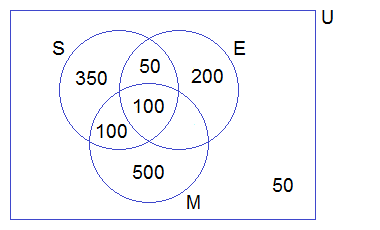Example 5: In a group of students, 25 study computer, 28 study Health, 20 study Mathematics, 9 study Computer only, 12 study Health only, 8 study Computer and Health only and 5 students study Health and Mathematics only.

(i)        Draw a Venn diagram to illustrate the above information.

(ii)     Find how many students study all the subjects.

(iii)  How many students are there altogether?

Solution:

Let C, H, and M be the set of students who study Computer, Health, and Mathematics respectively.

n(C) = 25

n(H) = 28

n(M) = 20

no(C) = 9

no(H) = 12

no(C∩H) = 8

no(H∩M) = 5

Filling the above information in Venn-diagram, we getFrom the above Venn-diagram,

n(C∩H∩M) + 8 + 12 + 5 = n(H)

or,     n(C∩H∩M) + 25 = 28

or,     n(C∩H∩M) = 28 – 25

or,     n(C∩H∩M) = 3

Again,

no(C∩M) + n(C∩H∩M) + 9 + 8 = n(C)

or,     no(C∩M) + 3 + 9 + 8 = 25

or,     no(C∩M) + 20 = 25

or,     no(C∩M) = 25 – 20

or,     no(C∩M) = 5

Again,

no(M) + no(C∩M) + n(C∩H∩M) + 5 = n(M)

or,     no(M) + 5 + 3 + 5 = 20

or,     no(M) + 13 = 20

or,     no(M) = 20 – 13

or,     no(M) = 7

(i)        Venn-diagram: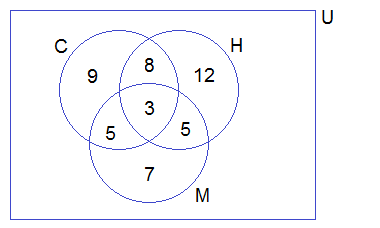(ii)     n(C∩H∩M) = 3 students study all subjects.

(iii)  From the above venn-diagram,

n(CHM)

= 9 + 8 + 3 + 5 + 12 + 5 + 7

= 49

There are 49 students altogether.

Example 6: In a group of people, 20 like milk, 30 like tea, 22 like coffee, 12 like coffee only, 6 like milk and coffee only,  2 like tea and coffee only and 8 like milk and tea only. Show these information in a Venn-diagram and find:

(i)        How many like at least one drink?

(ii)     How many like exactly one drink?

Solution:

Let the set of people who like milk, tea, and coffee be M, T, and C.

n(M) = 20

n(T) = 30

n(C) = 22

no(C) = 12

no(M∩C) = 6

no(T∩C) = 2

no(M∩T) = 8

Filling the above information in the Venn-diagram, we getFrom the above Venn-diagram,

n(M∩T∩C) + 6 + 12 + 2 = n(C)

i.e.     n(M∩T∩C) + 20 = 22

or,     n(M∩T∩C) = 22 – 20

or,     n(M∩T∩C) = 2

Again,

no(M) + 6 + n(M∩T∩C) + 8 = n(M)

i.e.     no(M) + 6 + 2 + 8 = 20

or,     no(M) + 16 = 20

or,     no(M) = 20 – 16

or,     no(M) = 4

Again,

no(T) + 2 + n(M∩T∩C) + 8 = n(T)

i.e.     no(T) + 2 + 2 + 8 = 30

or,     no(T) + 12 = 30

or,     no(T) = 30 – 12

or,     no(T) = 18

Venn-diagram,From the venn-diagram,

(i)        n(MCT)

= 4 + 6 + 12 + 2 + 18 + 8 + 2

= 52

52 like at least one drink.

(ii)     no(M) + no(C) + no(T)

= 4 + 12 +18

= 34

34 like exactly one drink.

If you have any questions or problems regarding the Word Problems Based on Three Sets, you can ask here, in the comment section below.

1.In a survey among 100 people,50 liked coffee,30 liked milk,40 liked tea,20 liked coffee only,25 liked tea only,10 liked tea and coffee and 5 liked all tea, coffee and milk.using the Venn diagram,find the number of people who liked neither of these.

1.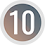Here is the solution to your problems,
[image src=" https://1.bp.blogspot.com/-5CpwLMYeUfI/YLxWRvfHgQI/AAAAAAAAJCQ/uvCnTcLPmUwl6pjX1mZ8zapXMMTwUBRcwCLcBGAsYHQ/s16000/Comment%2BSolution%2B1.png"/]
Comment here if you have more problems regarding the Word Problems Based on Three Sets.

2.In a group of students,20 study account,21 study maths,18 study history,7 study account only,10 study math only,6 study account and maths only and 3 study math and history only.if each student study at least one of the three subject,how many students study all the subject? How many students are there altogether?

1.Here is the solution to your problems,
[image src=" https://1.bp.blogspot.com/-v_RnnYLpy68/YLxWbakaXQI/AAAAAAAAJCU/ECRW98m3xT8G2sU62ybFhPwYXUIig9XqQCLcBGAsYHQ/s16000/comment%2Bsolution%2B2.png "/]
Comment here if you have more problems regarding the Word Problems Based on Three Sets.

3.In a school 20%students passed in all subjects(Maths,English and Dance),46% failed in maths,57% failed in English 42% failed in Dance,35% failed in maths and English,25% failed in English and Dance,15% failed in Dance and maths.What percentage of the studenta failed in all the three subjects ?

1.Here is the solution to your problems,

Solution:
Let the students who failed in Math, English and Dance be M, E and D respectively.
Passed in all subjects = 20%
∴ Failed in at least one subject = n(M∪E∪D) = 100 – 20% = 80%
n(M) = 46%
n(E) = 57%
n(D) = 42%
n(M∩E) = 35%
n(E∩D) = 25%
n(D∩M) = 15%
n(M∩E∩D) = ?

We know,
n(A∩B∩C)
= n(M∪E∪D) – n(M) – n(E) – n(D) + n(M∩E) + n(E∩D) + n(D∩M)
= 80 – 46 – 57 – 42 + 35 + 25 + 15%
= 10%

∴ 10% students failed in all three subjects.

Comment here if you have more problems regarding the Word Problems Based on Three Sets.

4.there are 100 cars in a car park. 60 of them are black, 40 of them are SUV's, 20 of the SUV's are automatic, the rest are manual. 15 of the manual SUV's are not black, 10 of the manual cars are not black, nor are they an SUV, 25 of the automatic cars are not an SUV, 41 of the black cars are not an SUV. How many automatic cars are there?

1.Here is the solution to your problems,
[image src="https://1.bp.blogspot.com/-5SFg01VbSDg/YOV08di9WgI/AAAAAAAAJbk/hQh09-tgLmAHZzQPWpFXS6GrmA0rDZRgACLcBGAsYHQ/s16000/comment%2Bsolution%2B3.png"/]
Comment here if you have more problems regarding the Word Problems Based on Three Sets.

5.In a class of 140 students, 80 offered mathematics, 72 offered chemistry and 68 offered physics, 40 offered mathematics and chemistry, 35 offered mathematics and physics while 30 offered chemistry and physics. How many students offered all three

1.Solution: Here,
Let the set of students who offered mathematics, Chemestry and Physics be M, C and H respectively.
n(U) = 140 = n(M∪C∪P)
n(M) = 80
n(C) = 72
n(P) = 68
n(M∩C) = 40
n(M∩P) = 35
n(P∩C) = 30
n(M∩C∩P) = ?

We know,
n(M∩C∩P)
= n(M∪C∪P) – n(M) – n(C) – n(P) + n(M∩C) + n(M∩P) + n(P∩C)
= 140 – 80 – 72 – 68 + 40 + 35 + 30
= 25

∴ 25 students offered all three subjects. Ans.

6.7.In a class of 240 students, a survey was conducted, 120 students had applied for university K, 100 students applied for university A and 96 students applied for university B. 42 students applied for both K and A, 24 applied for both K and B, 36 applied for both B and A while 16 applied neither of the aforementioned universities. Find
(a) number of students that applied for all the universities
(b) number of students that applied for at least two of the universities
(c) number of students that applied at most two universities
(d) number of students that applied for K but not A

1.Solution: Here,
n(U) = 240
n(K) = 120
n(A) = 100
n(B) = 96
n(K∩A) = 42
n(K∩B) = 24
n(A∩B) = 36
n(K∪A∪B)c = 16
We know,
n(A∪B∪C) = n(U) – n(K∪A∪B)c = 240 – 16 = 224
i.e. n(K) + n(A) + n(B) – n(K∩A) – n(K∩B) – n(A∩B) + n(K∩A∩B) = 224
or, 120 + 100 + 96 – 42 – 24 – 36 + n(K∩A∩B) = 224
or, 214 + n(K∩A∩B) = 224
or, n(K∩A∩B) = 224 – 214 = 10

a. No. of students who applied for all the universities = n(K∩A∩B) = 10
b. No. of students who applied at least two universities = n(K∩A∩B) + n(K∩A) + n(K∩B) + n(A∩B)
= 10 + 42 + 24 + 36
= 112
c. No. of students who applied at most two universities = n(K∪A∪B) – n(K∩A∩B)
= 224 – 10
= 214
d. No. of students who applied K but not A = n(K) – n(A)
= 120 – 100
= 20

2.Can you recheck answers of b and d

8.The following analysis shows how students from various departments registered for 2018/2019 academic session from the college of social and management science in Tai Solarin University of education. 10 from economics only, 150 from economics and geography, 90 from economics but not political science, 210 from geography, 120 from political science and geography, 180 from economics. If the total of students registered for courses is 250, determine the number of students who registered for I. Political science ii. Political science and geography but not economics iii. Economics and political science but not geography

1.Solution: Here,
no(E) = 10
n(E∩G) = 150
n(E – P) = 90
n(G) = 210
n(P∩G) = 120
n(E) = 180
n(U) = 250
no(E∩P)= n(E)-no(E) + n(E∩G) = 180 – (10 + 150) = 180 – 160 = 20
no(E∩G) = n(E – G) – no(E) = 90 – 10 = 80
n(E∩P∩G) = n(E) – (20 + 10 + 80) = 180 – 110 = 70
no(P∩G) = n(P∩G) - n(E∩P∩G) = 120 – 70 = 50
no(P) = n(U) – [n(G) + 10 + 20] = 250 – [210 + 10 + 20] = 250 – 230 = 20
n(P) = 20 + 70 + 50 + 20 = 160
(i) Political science = n(P) = 160
(ii) Political science and geography but not economics = no(P∩G) = 50
(iii) Economics and political science but not geography = no(E∩P)= 20

2.I believe there is a mistake where u found no(P)[it's 250-240]

9.Of 497 girls reading three newspapers 26 girls read the guardian and the vanguard,50 girls read sun and the vanguard, 38 girls read the guardian and the sun,and 11 read all the three newspapers.it was found that equal numbers read the sun,the guardian and the vanguard.(a) how many read only sun? (b) how many read only tee vanguard (c) how many read only one of these papers?

10.Of 1000 students applying for admission into faculty of physical sciences,Nnamdi Azikiwe University,Awka. 200 applied to read statistics, 240 applied to read chemistry and 250 applied to read computer sciences.97 applied to read statistics and chemistry only,while 64 applied to read chemistry and computer sciences only, 60 applied to read statistics and computer sciences only.if 570 students do not apply to any of these departments, how many students applied (a) only into the department of chemistry? (b)Only into one of these departments? (c) the three departments?

11.In a survey of people 48 liked Nepali film 40 liked English film 31 like Hindi film 19 liked nepali and hindi film 13 liked Hindi and English 11 liked Nepali only and 21 people were found not interested in any film 25 liked Nepali and English film find (a) how many liked all three films (b)how many were asked question ?

12.In a recent pool of 500 men and 500 women,it was observed that a total of 650 were married.of those married,275 were men and 500 claimed to be happy. Out of 750 claimed to be happy. 400 were men of which 200 were married. Represent this information in a venn diagram and find
(a)the number of married people who are happy
(b)the number of unmarried people who are not happy

1.Pls ineed Solution of this problem

13.The pandemic strikes and a subset of the 1000 students begin exhibiting symptoms of COVID, including fever, cough, and loss of smell. When the medical center screened the 200 symptomatic students, they found 150 students had a fever, 25 students had a cough, 25 students had loss of smell. In addition, 20 students appeared with fever and cough, 10 students showed cough and loss of smell, 5 students had fever and loss of smell. Calculate the number of students exhibiting all three symptoms: fever, cough, and loss of smell.

1.Solution: Here,
Let the set students having symptoms Fever, Cough and Loss of smell be F, C and L.
n(F∪C∪L) = 200
n(F) = 150
n(C) = 25
n(L) = 25
n(F∩C) = 20
n(C∩L) = 10
n(F∩L) = 5
n(F∩A∩M) = ?

n(F∩C∩L)
= n(F∪C∪L) – n(F) – n(C) – n(L) + n(F∩C) + n(C∩L) + n(F∩L)
= 200 – 150 – 25 – 25 + 20 + 10 + 5
= 35

All three symptoms = n(F∩C∩L) = 35

2.Thank you so much.

3.But as n(F)+n(C)+n(L)=n(FUCUL)
Aren't these sets disjoint and hence there can't be any intersection

14.London School of Business has matriculated 1000 Business graduates for the fall semester in 2017. Among them, 340 students have Finance, 320 students have Accounting, and 300 students have Management as their major in maters program. Also, some graduates have more than one concentration in their graduate program. Though, 100 students have gone in for all the three majors, it was inevitable from the chronicling by the dean office that 180 students have both finance and Accounting, 190 students have both Finance and Management, and 200 students have both accounting and Management as their major. Find out the number of students who has contrary close mental application with at least one of these three areas.

1.Solution: Here,
Let the set students having Finance, Accounting and Management be F, A and M.
n(U) = 1000
n(F) = 340
n(A) = 320
n(M) = 300
n(F∩A∩M) = 100
n(F∩A) = 180
n(F∩M) = 190
n(A∩M) = 200

n(F∪A∪M)
= n(F) + n(A) + n(M) - n(F∩A) - n(F∩M) - n(A∩M) + n(F∩A∩M)
= 340 + 320 + 300 – 180 – 190 – 200 + 100
= 490

At least one subjects = n(F∪A∪M) = 490

15.Please i need a solution to this question
A newspaper agent sells three papers, tge times,punch and herald,70 customers buy the times newspaper, 60 buy the punch and 50 buy the herald, 17 buy times and punch, 15 buy punch and herald and 16 buy the herald and times while 3 customers buy all three. Determine the number of customers

1.Solution: Here,
Let the set of customers buying tge times, punch and herald be T, P and H.
n(T) = 70
n(P) = 60
n(H) = 50
n(T∩P) = 17
n(P∩H) = 15
n(H∩T) = 16
n(T∩P∩H) = 3

By using formula,

n(T∪P∪H)
= n(T) + n(P) + n(H) – n(T∩P) – n(P∩H) – n(H∩T) + n(T∩P∩H)
= 70 + 60 + 50 – 17 – 15 – 16 + 3
= 135

∴ No. of customers = n(T∪P∪H) = 135

16.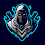In a survey, people were asked what types of movies they like. It was found the 75 liked Nepali movies, 60 liked English, 40 liked Hindi, 35 liked Nepali and English 30 liked Nepali and Hindi, 20 liked Hindi and English. 10 liked all three and 2 people were found not interested in any types of movies. (i) How many people did not like only Hindi films?

(ii) How many people did not like only Nepali or Hindi films?

Can I Get it's Solutions

1.Solution:
Let the set of people who like Nepali, English and Hindi be N, E and H.
n(N) = 75
n(E) = 60
n(H) = 40
n(N∩E) = 35
n(N∩H) = 30
n(H∩E) = 20
n(N∩E∩H) = 10
n(N∪E∪H)c = 2

no(N∩E) = n(N∩E) - n(N∩E∩H) = 35 – 10 = 25
no(H∩E) = n(H∩E) - n(N∩E∩H) = 20 – 10 = 10

(i) No. of people who did not like only hindi film = no(N∩E) = 25
(ii) No. of people who did not like only Nepali or Hindi films = no(N∩E) + no(H∩E) = 25+ 10 = 35

17.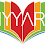Mathematics is a subject that is taught to all the students in schools. Many students find it very difficult to understand mathematics, due to which they fall behind. If you want to be good at maths then you can take Ziyyara’s 1-to-1 online tuition where experienced online tutors help your kids to maths problem solver.

18.In a survey of people, 48 liked Nepali films, 40 liked English films, 31 liked Hindi films. 19 liked Nepali and Hindi films, 13 liked Hindi and English, 11 liked Nepali only and 21 people were found not interested in any film. Find:

(i) How many people liked all the three films?
(ii) How many people were asked this questions?

1.Solution:
Let the set of people who like Nepali, English and Hindi films be N, E and H.
n(N) = 48
n(E) = 40
n(H) = 31
n(N∩H) = 19
n(H∩E) = 13
no(N) = 11
n(N∪E∪H)c = 21
(i) n(N∩E∩H) = ?
(ii) n(U) = ?

n(E∪H) = n(E) + n(H) – n(E∩H)
= 40 + 31 – 13
= 58
n(N∪E∪H) = no(N) + n(E∪H)
= 11 + 58
= 69
n(U) = n(N∪E∪H) + n(N∪E∪H)c
= 69 + 21
= 90
n(N∩E) is should be given to find the value of n(N∩E∩H).

19.Plz solve it fast asap

20.In a survey of 100 outpatients who reported at a hospital one day, it was found that 70 complained of fever , 50 complained of stomach ache and 30 were injured. All patients had at least one of the complaints and 44 had exactly two of the three complaints. How many patients had all the three complaints?

1.Solution:
Let the set of patients of fever, stomach ache and injured be F, S and I.
n(U) = 100
n(F) = 70
n(S) = 50
n(I) = 30
no(F∩S) + no(S∩I) + no(F∩I) = 44
n(F∩S∩I) = x (let)

All patients had at least one of the complaints, therefore,
n(F∪S∪I) = n(U)
i.e.
n(F) + n(S) + n(I) – n(F∩S) – n(S∩I) – n(F∩I) + n(F∩S∩I) = 100
or, n(F)+n(S)+n(I) - [no(F∩S)+n(F∩S∩I)] - [no(S∩I)+n(F∩S∩I)] - [no(F∩I)+n(F∩S∩I)]+n(F∩S∩I) = 100
or, n(F)+n(S)+n(I) - no(F∩S) - n(F∩S∩I) - no(S∩I) - n(F∩S∩I) - no(F∩I) - n(F∩S∩I) + n(F∩S∩I) = 100
or, n(F)+n(S)+n(I) - no(F∩S) - no(S∩I) - no(F∩I) - 2n(F∩S∩I) = 100
or, n(F)+n(S)+n(I) - [no(F∩S) + no(S∩I) + no(F∩I)] - 2n(F∩S∩I) = 100
or, 70 + 50 + 30 - 44 - 2x = 100
or, 106 - 2x = 100
or, - 2x = 100 – 106
or, - 2x = - 6
or, x = -6/-2
or, x = 3

∴ n(F∩S∩I) = 3
∴ 3 patients had all three complaints.

21.In a survey of 78 students of class X,the ratio of the number of students who like Math to the number of students who like Science is 3:4. If 15 students do not like both the subjects and 35 students like both subjects.
i) Find the number of students who prefer only Math?

1.Plz solve it fast

2.Solution:
Let the set of students who like Math and Science be M and S.
n(U) = 78
n(M):n(S) = 3:4
∴ Let, n(M) = 3x and n(S) = 4x
n(M∩S) = 35
n(M∪S)c = 15
no(M) = ?

We know,
n(M∪S) = n(U) - n(M∪S)c = 78 – 15 = 63
i.e. n(M) + n(S) - n(M∩S) = 63
or, 3x + 4x – 35 = 63
or, 7x = 63 + 35
or, x = 98/7
or, x = 14

∴ n(M) = 3x = 3×14 = 42
∴ no(M) = n(M) - n(M∩S) = 42 – 35 = 7
∴ 7 students prefer math only.

22.in Lagos State polytechnic, A class of 22 business administration student take or more of GNS,MTH,CTZ courses. 12 takes MTH, 8 takes CTZ, 7 takes GNS, nobody takes MTH and GNS, 4 student takes MTH and CTZ. draw a venn diagram to illustrate information below. (1) how many students takes both GNS and CTZ (2) how many student take CTZ (3) how many student take exactly one course (4) how many student take exactly 2 courses

1.Here is the solution to your problem,

23.There are 98 language students.out of this number, 92 own a phone or a calculator or a laptop.31 students own a phone, 73 own a calculator and 25 own a laptop. it is known that 15 own both a calculator and laptop, 12 own both phone and and a laptop and 13 own both a calculator and a phone.Represent the information on a venn diagram. Use the diagram to calculate the number of students who own;
A. A phone, a calculator and a laptop
B. A phone only or a calculator only or a laptop only.

1.Here is the solution to your problem,

24.In a class of 60 students,47 study mathematics,33 study mathematics and physics,31study mathematics and Chemistry, 29 study physics and Chemistry and 20 study all the three subjects. If the number of students who study only physics is equal to that of those who study only chemistry.
B illustrate the given information on a Venn diagram
C. Find the number of students who study
I only physics
ii chemistry
iii only one subject

1.Here is the solution to your problem,

25.26.279 courses were offered online, 68 took Japanese, 90 took Arabic and 57 took Chinese. While 29 took both Japanese and Arabic, 25 took both Japanese and Chinese, 24 took both Arabic and Chinese and 14 took all. How many took only Japanese, Arabic and Chinese, respectively?

1.Solution:
Let the set of Japanese, Arabic and Chinese be J, A and C.
n(U) = 279
n(J) = 68
n(A) = 90
n(C) = 57
n(J∩A) = 29
n(J∩C) = 25
n(A∩C) = 24
n(J∩A∩C) = 14
no(J) = ?, no(A) = ?, no(C) = ?

By Formula,
no(J) = n(J)-n(J∩A)-n(J∩C)+n(J∩A∩C) = 68-29-25+14 = 28
no(A) = n(A)-n(J∩A)-n(A∩C)+n(J∩A∩C) = 90-29-24+14 = 51
no(C) = n(C)-n(J∩C)-n(A∩C)+n(J∩A∩C) = 57-25-24+14 = 22

27.A number of artists were asked to identify their favourite video the results are summarized below

62 appreciate Africa,40 appreciate American, 5 appreciate asian,2 appreciate african nd American nd Asian,3 appreciate American nd Asian, 20 appreciate african nd American, 42 appreciate Africa nd neither of the two.12 appreciate none of the three videos.

How many surveyed ?
How many do appreciate Asian?
How many appreciate Africa nd Asian?
How many appreciate exactly one of the three videos.

1.Here is the solution to your problem,

28.A group of tourists on an expedition tour were asked to indicate which of the following languages; French,English or Arabic they could speak.
30 of them could speak French
25 could speak English and
15 could speak Arabic
12 could speak two of the 3 languages. Of those who could speak two languages,6 could speak Arabic and 2 of them could not speak English. However 7 of them could speak French and english. Find;

A-The total number of tourist in the group
B-The number of tourists who could speak only one of the 3 languages

1.Here is the solution to your problem,

29.In a survey concerning the habit of watching T.V, it was found that 60% people watch program A, 50% watch program B, 50% watch program C, 30% watch program A and B, 20% watch program B and C,30% watch program C and A,and 10% do not watch any program. Find what percent watch program A,B,C?

1.Solution:
Here,
n(U) = 100%
n(A) = 60%
n(B) = 50%
n(C) = 50%
n(A∩B) = 30%
n(B∩C) = 20%
n(C∩A) = 30%
n(A∪B∪C)c = 10%
n(A∩B∩C) = ?

n(A∪B∪C) = n(U) - n(A∪B∪C)c = 100 – 10% = 90%
n(A∩B∩C) = n(A∪B∪C) – n(A) – n(B) – n(C) + n(A∩B) + n(B∩C) + n(A∩C)
= 90 – 60 – 50 – 50 + 30 + 20 + 30
= 170 – 160
= 10%

∴ 10% watch program A, B, C.

30.In a survey of 500 families, the that read recent issues of a certain monthly magazine were found to be : March only 90; March but no February 115; March and January 40; March 130; January 240,January and February 40; none of the three months 120.With the help of set theory, find the following :
1. How many did read February issue?
2. How many did read two consecutive issues?
3. How many did read the January issue, if and only if they did not read the February issue?
4.How many did read the March and February issues but not the January issue?

1.Here is the solution to your problem,

31.50 biochemistry first year students had a choice of beans,plantain,and rice for analysis.21 of them analysed beans,24 analysed plantain and 18 analysed rice.3 analysed beans only,9 analysed plantain only and 2 analysed rice only.Only 5 analysed all the three food items.
(A)Draw a venn diagram to illustrate the above information.
(B)Use your diagram to find the number of students who analysed
(i)Rice and beans only
(ii)None of the three food items

1.Here is the solution to your problem,

32.Mr.Arefin has 165 workers in process x, 110 workers in process y and 97 workers in process z in his firm.Out of these workers, 281 workers are skilled in the activities of x and/or y, 269 workers are skilled in the activities of y and/or z, 241 workers are skilled in the activities x and/or z. However 44 workers are unskilled. Accounting Department of these firm has informed that the average monthly earning of different type of workers are as follows: workers who are skilled in the activities of at least two processes : Tk.3500; workers who are skilled in the activities of any one process:2500; workers who are unskilled :Tk.1500. Find the monthly amount of total earnings of all workers of the firm.

33.In a competition, a school awarded medals in different categories. 46 medals in dance, 12 medals in dramatics and18 medals in music. If these medals went to a total of 45 persons and only 4 persons got medals in all three categories, how many received medals in exactly two of these categories

34.In a class of 77 students all but 7 went on at one of the college visits arranged to Accra, Kumasi, and Takoradi. 11 went to both Accra and Kumasi but not Takoradi, 3 students went to both Accra and Takoradi but not Kumasi. 5 students went to all three cities. Altogether the number of students who visited Accra, Kumasi, Takoradi were 39, 35 and 20 respectively.
a. Illustrate the above information in a Venn diagram.
b. Calculate the number of students who visited Kumasi and Takoradi.
c. How many students visited only one city

1.Here is the solution to your problem,

35.The following were the result of a survey of 150 people that was conducted to find out the type of fish they like among chambo, mcheni and usipa. 80 people like chambo, 60 like mcheni and 35 like usipa; 7 like chambo and usipa only, 10 like chambo and mcheni only and 5 like mcheni and usipa only; 33 do not like any of the three types. (a) present the information in the a venn diagram. (b) use the venn diagram to who like all three types of fish

1.36.Salaamu'alaykum wa rahmatoulie wa barakaatuhu! I really need urgent help on this set problem.

In a class of 80 students, 53 study Art, 60 study Biology, 36 study Art and Biology, 34 study Art and Chemistry, 6 study Biology only and 18 study Biology but not Chemistry. Illustrate the information on a Venn diagram. Determine the number of students who study:
i) Art only
ii) Chemistry

1.Here is the solution to your problem,

37.Of 497 girls reading three newspapers 26 girls read the Guardian and
the Vanguard, 50 girls read the Sun and the Vanguard, 38 girls read the
Guardian and the Sun, and 11 read all the three three newspapers. It was
found that equal numbers read the Sun, the Guardian and the Vanguard.
(a) How many read only the Sun? (b) How many read only the
Vanguard? (c) How many read only one of these papers?

1.Here is the solution to your problem,

38.Student test results on three modules; Elementary Business Mathematics, Basic Book keeping and
Basic Computer Application show that 20 passed Basic Computer Application 5 passed all three
subjects, 12 passed Elementary Business Mathematics and Basic Book keeping and 16 passed
Elementary Business Mathematics and Basic Computer Application. Each student passed at least
two modules.
i) Draw a well labeled Venn diagram to represent these results.
ii) How many students passed Basic Book keeping and Basic Computer Application?
iii) How many students did the test?

39.In a class of 30 students.20 like football and 16 like hockey..10 like volleyball and hockey
1.illustrate this information on a venn diagram
2.find at least two games

40.A member of Pensa group were asked to indicate which of the Ghanaian language Twi,Ga,Ewe they could speak .It was found that 30 students could speak Twi , 23 could speak Ga and 17 could speak Ewe . 12 students could speak two of the three languages,5 could not speak Ewe and 3 could not speak Ga .Howerever every member could speak one of the 3 languages.
I.How many members were there in the group
II. How many members can speak only one of the language

1.There seems to have some error in the given values, because
n(U) = n(T∪G∪E) = 17 + 5 = 23 + 3 (False)

41.42.In an examination, 53% students passed in Mathematics, 61% passed in Economics , 60% passed in statistics, 24% passed in Mathematics and Economics, 35% in Economics and Statistics, 27% in Mathematics and Statistics and 5% passed in noneof the subjects. How many students passed in all the three subjects ?How many students failed in exactly one subject?

1.Solution:

Let, the set of students who passed in Mathematics, Economics and Statistics be M, E, and S respectively.

n(U) = 100%
n(M) = 53%
n(E) = 61%
n(S) = 60%
n(M∩E) = 24%
n(E∩S) = 35%
n(M∩S) = 27%
n(M∪E∪S)c = 5%

n(M∪E∪S) = n(U) - n(M∪E∪S)c = 100 – 5% = 95%

Passed in all three subjects,
= n(A∩B∩C)
= n(A∪B∪C) – n(A) – n(B) – n(C) + n(A∩B) + n(B∩C) + n(A∩C)
= 95 – 53 – 61 – 60 + 24 + 35 + 27%
= 7%

no(M∩E) = n(M∩E) – n(M∩E∩S) = 24 – 7 = 17%
no(E∩S) = n(E∩S) – n(M∩E∩S) = 35 – 7 = 28%
no(M∩S) = n(M∩S) – n(M∩E∩S) = 27 – 7 = 20%

Failed in exactly one subject,
= no(M∩E) + no(E∩S) + no(M∩S)
= 17 + 28 + 20%
= 65%

43.in a survey number of student who like only one vitamin is equal to twice number of students who like only two vitamin if two student like all three vitamin A B and C and the number of students who don't like only two vitamin are 50 find the total number of student

44.The school is offering special courses in two different subjects: Mathematics and Science. Students are free to enroll in special courses from both subjects based on their interests and academic requirements.Here's the enrollment data:- Among the students, 120 have chosen at least one Mathematics course.- Among the students, 90 have chosen at least one History course.- A total of 150 students are enrolled. How many students have chosen both Mathematics and Science courses? How many students have only chosen Math? How many students have not chosen any course?